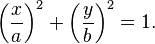Batman and Superman Maths

Wolfram Alpha is an incredibly powerful mathematical tool – which has been developed to allow both complex calculations and data analysis. It is able to generate images like that shown above, of the Batman logo. What’s really impressive however is that you can see the underlying graph input that would generate this image:At first glance this look indecipherable – but we can actually understand this a little better by breaking these inequalities down and looking at the individually.The first inequality defines the area inside an ellipse.  All ellipses have a general formula:$\left(\frac{x}{a}\right)^2 + \left(\frac{y}{b}\right)^2 = 1.$

In our inequality, the a simply stands for an arbitrary constant (because the Batman logo has no scale).  To keep things simple we can set a = 1.  This gives an equation:which generates the ellipse:When we now make this the inequality:Then this simply has the effect of shading in the area contained within the ellipse.  So, comparing this to the original Batman shape we can see that the ellipse we have drawn forms the wings of the logo.

Next, let’s look at the next inequality:Which, if we again choose a = 1 for simplicity, we will getwhen the part of this graph which is greater or equal to 4.

I’m not going to go through each part – as that would take too long!  Let’s look at one more inequality though:This will generate the part of the graph that looks like:This will form part of the Batman logo cape.

Superman LogoNow if you thought that was hard, have a look at the inequalities needed for the Superman logo above:Now this really is almost indecipherable!  I can at least explain what the min(a,b) means.  For example, say we had:

y = min(cosx,sinx)

This would simply mean that for any x value, I would find out what cosx was equal to, find out what sinx was equal to, and then plot the smallest value as my y value.  For example, when x = 0, I would have cos(0) = 1 and sin(0) = 0.  So I choose my y value as 0 when x = 0.  Plotting this graph would give:

Which is an interesting periodic function that shares some of the features of the regular trig graphs.  Anyway, the real Superman inequality is much harder than this – and demonstrates just how powerful Wolfram Alpha is.IB RevisionIf you’re already thinking about your coursework then it’s probably also time to start planning some revision, either for the end of Year 12 school exams or Year 13 final exams. There’s a really great website that I would strongly recommend students use – you choose your subject (HL/SL/Studies if your exam is in 2020 or Applications/Analysis if your exam is in 2021), and then have the following resources:The Questionbank takes you to a breakdown of each main subject area (e.g. Algebra, Calculus etc) and each area then has a number of graded questions. What I like about this is that you are given a difficulty rating, as well as a mark scheme and also a worked video tutorial.  Really useful!The Practice Exams section takes you to ready made exams on each topic – again with worked solutions.  This also has some harder exams for those students aiming for 6s and 7s and the Past IB Exams section takes you to full video worked solutions to every question on every past paper – and you can also get a prediction exam for the upcoming year.

I would really recommend everyone making use of this – there is a mixture of a lot of free content as well as premium content so have a look and see what you think.